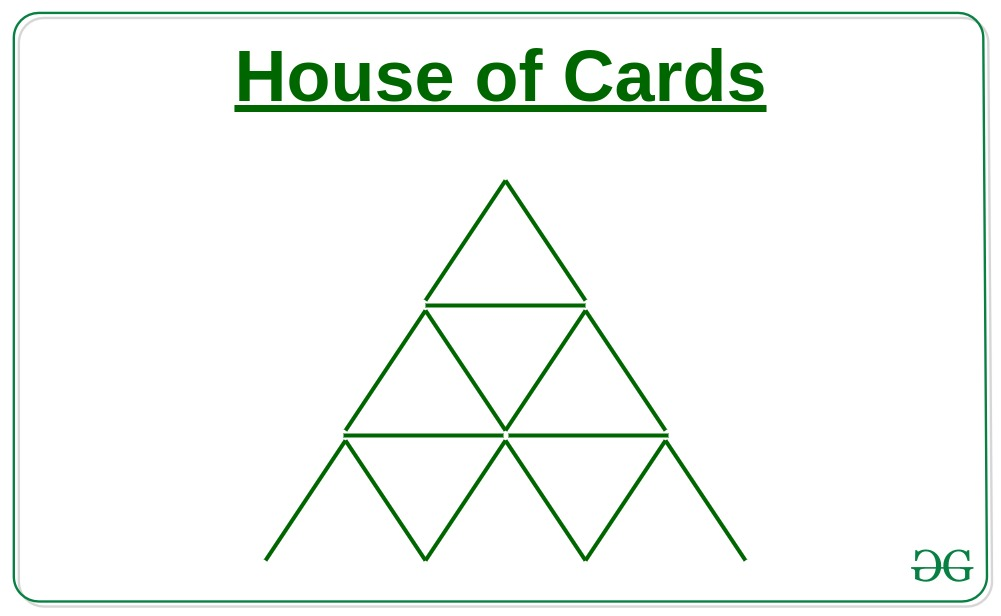# Count triangles required to form a House of Cards of height N

• Difficulty Level : Medium
• Last Updated : 17 Mar, 2021

Given a number N, the task is to count the number of triangles required to form a House of Cards of N levels.

Examples:

Input: N = 3
Output: 13
Explanation:From the above image, the following observations can be made:
Count of triangles of unit 1 = 9 (6 non-inverted triangles and 3 inverted triangles)
Count of triangles of unit 2 = 3
Count of triangles of unit 3 = 1
Therefore, total number of triangles = 6 + 3 + 3 + 1 = 13

Input: N = 2
Output: 5

Approach: The required number of triangles required to form a House of Cards of N levelscan be calculated by the formula:Illustration:

For N = 3
Count of triangles = 3 * (3 + 2) * (6 + 1) / 8 = 13

For N = 2
Count of triangles = 2 * (2 + 2) * (4+ 1) / 8 = 5

Below is the implementation of the above approach:

## CPP

 // C++ implementation of the// above approach #include using namespace std; // Function to find the// number of trianglesint noOfTriangles(int n){    return floor(n * (n + 2)                 * (2 * n + 1) / 8);} // Driver Codeint main(){    int n = 3;    // Function call    cout << noOfTriangles(n) << endl;    return 0;}

## Java

 // Java implementation of the// above approach import java.lang.*; class GFG {     // Function to find number of triangles    public static int noOfTriangles(int n)    {        return (n * (n + 2) * (2 * n + 1) / 8);    }     // Driver Code    public static void main(String args[])    {        int n = 3;        // Function call        System.out.print(noOfTriangles(n));    }}

## Python3

 # Python3 implementation of# the above approach # Function to find required# number of trianglesdef noOfTriangles(n):    return n * (n + 2) * (2 * n + 1) // 8  # Driver Coden = 3 # Function callprint(noOfTriangles(n))

## C#

 // C# implementation of the// above approachusing System; class GFG {    // Function to find number of triangles    public static int noOfTriangles(int n)    {        return (n * (n + 2) * (2 * n + 1) / 8);    }     // Driver Code    public static void Main(String[] args)    {        int n = 3;        Console.Write(noOfTriangles(n));    }}

## Javascript

 

Output:

13

Time Complexity: O(1)
Auxiliary Space: O(1)

My Personal Notes arrow_drop_up